Worksheet: What is Long, What is Round?

# Worksheet: What is Long, What is Round? | Mathematics for Class 2: NCERT

 1 Crore+ students have signed up on EduRev. Have you?

Q.1. Circle the 3D shapes that stack.
(i)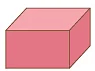(ii)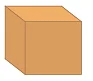(iii)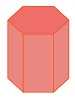(iv)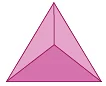(v)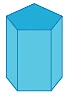(vi)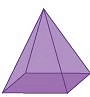Q.2. Pick the 3D shapes that slide.
(i)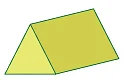(ii)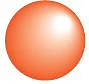(iii)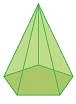(iv)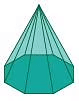(v)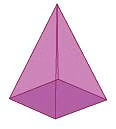(vi)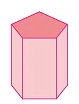Q.3. Find out the 3D shapes that roll
(i)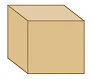(ii)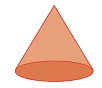(iii)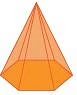(iv)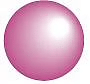(v)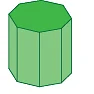(vi)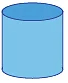Q.4. Fill in the blanks: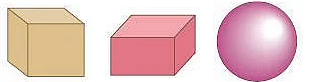(i) The cube and the cuboid each has _________ plane surfaces, _________ edges, and _________ vertices.
(ii) The cuboid has _________ pairs of identical plane surfaces.
(iii) All the surfaces of a cube are _________ to each other.
(iv) All the edges of a cube are _________.
(v) Opposite edges of a cuboid are _________.
(vi) A _________ has no vertex, and no edge.

Q.5.Write ‘true’ or ‘false’ for each statement as the case may be: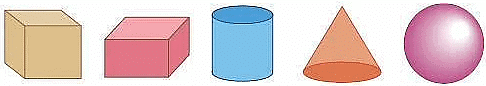(i) Every cuboid is a cube.
(ii) Every cube is a cuboid.
(iii) All faces of a cube are similar in shape and size.
(iv) All the faces of a cuboid are identical.
(v) The cube has 6 faces.
(vi) The base and bottom of a cylinder are identical.
(vii) A cone has no vertex.
(viii) A cylinder has two edges.
(ix) A sphere has no edge.
(x) A cone has two surfaces.

The document Worksheet: What is Long, What is Round? | Mathematics for Class 2: NCERT is a part of the Class 2 Course Mathematics for Class 2: NCERT.
All you need of Class 2 at this link: Class 2
FAQs on Worksheet: What is Long, What is Round? | Mathematics for Class 2: NCERT
 1. What is the difference between long and round objects?Ans. Long objects are those which have a greater length than their width, while round objects are those which are circular in shape and have no sharp edges. Examples of long objects include pencils, rulers, and chopsticks, while examples of round objects include balls, coins, and plates.
 2. Can an object be both long and round?Ans. No, an object cannot be both long and round as these are two distinct and mutually exclusive characteristics. An object can either be long or round, but not both at the same time.
 3. How do you measure the length of a long object?Ans. To measure the length of a long object, you can use a ruler or a tape measure. Simply place the ruler or tape measure alongside the object and read the measurement where the object ends. Make sure to measure from one end to the other and not just a part of the object.
 4. What are some examples of round objects that are not perfectly circular?Ans. Some examples of round objects that are not perfectly circular include eggs, fruit, and marbles. These objects may have slight variations in their shape, but they are still considered round because they lack sharp edges and have a curved surface.
 5. How can you determine if an object is long or round if it is not obvious?Ans. If an object's shape is not clearly long or round, you can look at its dimensions and compare its length and width. If the length is significantly greater than the width, the object is likely long. If the length and width are roughly the same, the object is likely round. Additionally, you can also look at the object's edges and surface to determine if it has any sharp edges or curves.

## Mathematics for Class 2: NCERT

34 videos|65 docs|21 tests

## Mathematics for Class 2: NCERT

34 videos|65 docs|21 tests

Track your progress, build streaks, highlight & save important lessons and more!(Scan QR code)

,

,

,

,

,

,

,

,

,

,

,

,

,

,

,

,

,

,

,

,

,

,

,

,

;